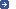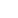– the intergovernmental organization through which Member States act together
on matters related to measurement science and measurement standards.
 Search facility:| Site map | News | Contact us> You are here: worldwide metrology > CGPM > outcomes > 11th meeting > Resolution 12Resolution 12 of the 11th CGPM (1960) Version françaiseSyst�me International d'Unit�s*The 11th Conférence Générale des Poids et Mesures (CGPM),

considering

• Resolution 6 of the 10th CGPM, by which it adopted six base units on which to establish a practical system of measurement for international use:
•  length metre m mass kilogram kg time second s electric current ampere A thermodynamic temperature degree Kelvin �K luminous intensity candela cd

• Resolution 3 adopted by the Comité International des Poids et Mesures (CIPM) in 1956,
• the recommendation adopted by the CIPM in 1958 concerning an abbreviation for the name of the system, and prefixes to form multiples and submultiples of the units,
• decides

1. the system founded on the six base units above is called the "Système International d'Unités";
2. the international abbreviation of the name of the system is SI;
3. names of multiples and submultiples of the units are formed by means of the following prefixes:

4.  Multiplying factor Prefix Symbol Multiplying factor Prefix Symbol 1 000 000 000 000 = 1012 tera T 0.1 = 10–1 deci d 1 000 000 000 = 109 giga G 0.01 = 10–2 centi c 1 000 000 = 106 mega M 0.001 = 10–3 milli m 1 000 = 103 kilo k 0.000 001 = 10–6 micro µ 100 = 102 hecto h 0.000 000 001 = 10–9 nano n 10 = 101 deca da 0.000 000 000 001 = 10–12 pico p

5. the units listed below are used in the system, without excluding others which might be added later.

Supplementary units

Derived units

 area square metre m2 volume cubic metre m3 frequency hertz Hz 1/s mass density (density) kilogram per cubic metre kg/m3 speed, velocity metre per second m/s angular velocity radian per second rad/s acceleration metre per second squared m/s2 angular acceleration radian per second squared rad/s2 force newton N kg � m/s2 pressure (mechanical stress) newton per square metre N/m2 kinematic viscosity square metre per second m2/s dynamic viscosity newton-second per square metre N � s/m2 work, energy, quantity of heat joule J N � m power watt W J/s quantity of electricity coulomb C A � s tension (voltage), potential difference, electromotive force volt V W/A electric field strength volt per metre V/m electric resistance ohmV/A capacitance farad F A � s/V magnetic flux weber Wb V � s inductance henry H V � s/A magnetic flux density tesla T Wb/m2 magnetic field strength ampere per metre A/m magnetomotive force ampere A luminous flux lumen lm cd � sr luminance candela per square metre cd/m2 illuminance lux lx lm/m2Reference:Comptes Rendus de la 11e CGPM (1960), 1961, p.87Note: The CGPM later abrogated certain of its decisions and extended the list of prefixes: The name and symbol for the unit of thermodynamic temperature were modified by the 13th CGPM in 1967 (Resolution 3). A seventh base unit, the mole, was adopted by the 14th CGPM in 1971 (Resolution 3). Further prefixes were adopted by the 12th CGPM in 1964 (Resolution 8), the 15th CGPM in 1975 (Resolution 10), and the 19th CGPM in 1991 (Resolution 4). The 20th CGPM in 1995 abrogated the class of supplementary units in the SI (Resolution 8). These are now considered as derived units. The 13th CGPM in 1967 (Resolution 6) specified other units which should be added to this list of derived units. In principle, the list is without limit. Modern practice is to use the phrase "amount of heat" rather than "quantity of heat", because the word quantity has a different meaning in metrology. Modern practice is to use the phrase "amount of electricity" ranther than "quantity of electrcity" (see above).The reader should note that the official version of this Resolution is the French text.Other Resolutions of the 11th CGPM (1960)Resolution 1Resolution 2Resolution 3Resolution 4Resolution 5Resolution 6Resolution 7Resolution 8Resolution 9Resolution 10Resolution 11Resolution 12Resolution 13Resolution 14Resolution 15Resolution 16BIPM - Pavillon de Breteuil F-92312 Sèvres Cedex FRANCE Disclaimer Privacy Policy Copyright © BIPM all rights reserved